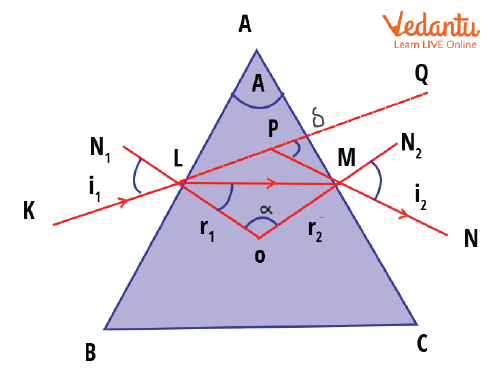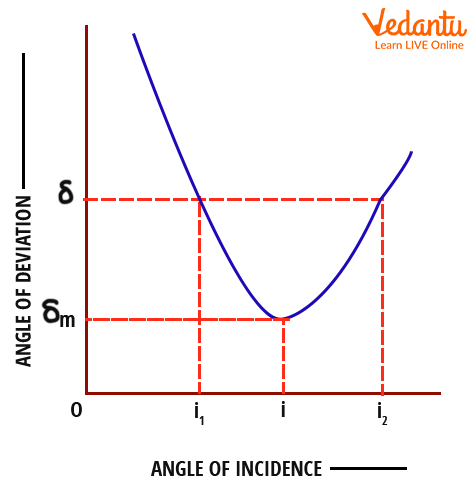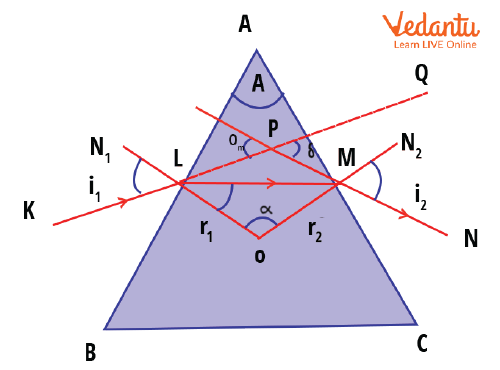Courses
Courses for Kids
Free study material
Free LIVE classes
More

# Angle of Deviation in Prism - Important Formula with Solved Problems for JEE## What is a Prism?

Last updated date: 01st Apr 2023
Total views: 81.6k
Views today: 1.57k

A prism can be defined as a wedge-shaped body that is made from a refracting medium. It is bound by two plane faces which are placed at an incline with each other at some angle. The two plane faces of the prism are called the refracting faces. The angle between the two faces is known as the angle of the prism. It is also known as the refracting angle. The faces of a prism can be parallelograms or rectangles without their bases. There are a variety of prisms and depending on the shape, the base of a prism could be a triangle, square, rectangle, or any n-sided polygon in general.

## What is The Angle of Deviation in Prism?

Before deriving the expression of the angle of deviation in the prism, it is important to understand what happens to a beam of light that is incident on one of the faces of the prism. A light that is incident on a surface of the prism undergoes refraction at both the surfaces of the prism and is deviated from the original path that was supposed to follow. There is a deviation in the path of the beam of light due to refraction from the faces of the prism. The angle of deviation is the angle between the original path that the beam should have followed and the deviated beam. It can also be defined as the angle through which the incident ray gets deviated when passing through a prism.Image: Angle of deviation in a prism

From the above diagram, we can see that a ray KL is incident on the face AB of the prism that we have. KL is incident on point L from where N1LO is the normal and i1 can be termed as the angle of incidence. Now the light will be refracted from this surface and since the refraction takes place from air to glass, the ray LM will bend towards the normal such that r1 will be the angle of refraction. Considering µ to be the refractive index of the material of the prism, we can write using Snell’s law,

$\mu=\dfrac {\sin {i_1}}{\sin {r_1}}$

Now the refracted ray LM will be incident on another face AC of the prism at point M. At this point, N2MO is normal, so r2 will be the angle of incidence. Now the refraction is taking place from the material to air, that is from a denser medium to a rarer medium. So the emergent ray MN will bend away from the normal and i2 will be the angle of emergence.

As discussed above, without the prism, the incident ray KL would have followed a straight path but due to the presence of the prism, it changes its path to PMN. So the angle QPN, or δ is the angle of deviation. It is the angle through which the incident ray deviates when passing through a prism.

From the elementary analysis of the angles, we can write,

\begin{align} &\delta=i_{1}-r_{1}+i_{2}-r_{2} \\ &\delta=i_{1}+i_{2}-\left(r_{1}+r_{2}\right) \end{align}

We can also write from triangle LOM,

$\alpha=180^{\circ}-\left(r_{1}+r_{2}\right)$

Also, we know that

$\angle A L O=\angle A M O=90^{\circ}$

In quadrilateral ALOM we can write,

\begin{align} &\angle A+\angle A L O+\alpha+\angle A M O=360^{\circ} \\ &\angle A+90^{\circ}+180^{\circ}-\left(r_{1}+r_{2}\right)+90^{\circ}=360^{\circ} \\ &\angle A=360^{\circ}-360^{\circ}+\left(r_{1}+r_{2}\right) \\ &\angle A=\left(r_{1}+r_{2}\right) \end{align}

Using this value of angle A we can write,

\begin{align} &\delta=i_{1}+i_{2}-A \\ &\delta+A=i_{1}+i_{2} \end{align}

The above equation shows the relationship between the angle of prism and the angle of deviation. This equation shows that the angle of deviation is dependent on the nature of the material as well as the angle of incidence.

## Minimum Angle of Deviation and Refractive Index

From experimental data, the curve given below has been plotted. The curve has been plotted between the angle of deviation and angle of incidence. One thing to be noted from the plot is that the angle of deviation has a minimum value $\delta_m$ only for one value of angle of incidence. This minimum value is known as the angle of minimum deviation. It is the angle of deviation when a ray of light passes through the prism with minimum deviation.Image: Plot between Angle of Deviation and Angle of incidenceImage: Angle of minimum deviation in prism

The above figure shows the prism in a minimum deviation position. Now if any plane mirror is placed in the path of the emergent ray, in this case, MN then the ray will retrace its original path. It will suffer a minimum deviation of $\delta_m$.

For the minimum deviation position, we have from the above graph,

$\angle i_{1}=\angle i_{2}$

We can also say,

$\angle r_{1}=\angle r_{2}=\angle r$

We can also write,

$\angle A L M=\angle L M A=90^{\circ}-\angle r$

This means that AL=LM and so, LM is parallel to BC.

From all this, we can infer that the ray which suffers minimum deviation passes symmetrically through the prism and is parallel to the base.

Now we can write,

$\angle A=\angle r_{1}+\angle r_{2}$

Since $\angle r_{1}=\angle r_{2}=\angle r$,

A = 2r or

$r=\dfrac{A}{2}$

And since $\angle i_{1}=\angle i_{2}$, we let them be i1. We can write,

\begin{align} &2 i_{1}=A+\delta m \\ &i_{1}=\dfrac{A+\delta m}{2} \end{align}

Now we know that,

$\mu=\dfrac {\sin {i_1}}{\sin {i_2}}$

From the above equations, we can write,

$\mu=\dfrac{\sin \left(\dfrac{(A+\delta m)}{2}\right)}{\sin \left(\dfrac{A}{2}\right)}$

This is the relationship between the angle of minimum deviation and the refractive index of the material of the prism.

## Numerical Examples on Angle of Deviation

Example 1: If the angle of minimum deviation of a light ray passing through a prism of refracting angle 60° is 30°, then find the refractive index of the prism.

Solution:

We know that,

$\mu=\dfrac{\sin \left(\dfrac{(A+\delta m)}{2}\right)}{\sin \left(\dfrac{A}{2}\right)}$

We have,

A=60° and $\delta_m$ = 30°

Substituting these values in the equation gives,

\begin{align} &\mu=\dfrac{\sin \left(\dfrac{60^{\circ}+30^{\circ}}{2}\right)}{\sin \left(\dfrac{60^{\circ}}{2}\right)} \\ &\mu=\dfrac{\sin 45^{\circ}}{\sin 30^{\circ}} \\ &\mu=1.414 \end{align}

So the refractive index of the material is 1.414.

Example 2: The refractive index of the material of a prism is 1.5. When the prism is placed in a minimum deviation position, the angle of incidence is 45º. Calculate the angle of prism and the angle of minimum deviation.

Solution:

We have  $\mu=1.5,~i=45^{\circ},~A=?,~\delta m=?$

We know that for minimum deviation,

\begin{align} &2 i=A+\delta_{m} \\ &2 \times 45^{\circ}=A+\delta_{m} \\ &90^{\circ}=A+\delta_{m} \end{align}

Also, we know that

$\mu=\dfrac{\sin \left(\dfrac{(A+\delta m)}{2}\right)}{\sin \left(\dfrac{A}{2}\right)}$

Substituting for  90° =  A +  $\delta_m$ we get,

\begin{align} &\sin \left(\dfrac{A}{2}\right)=\dfrac{\sin \left(45^{\circ}\right)}{1.5} \\ &A=2 \cdot \sin ^{-1}(0.471) \\ &A \approx 2 \cdot 28^{\circ} \\ &A \approx 56^{\circ} \end{align}

Substituting this value of A in  90° =  A +  $\delta_m$, we get,

\begin{align} &90^{\circ}-56^{\circ}=\delta_m \\ &34^{\circ}=\delta_m \end{align}

So the angle of the prism is 56° and the angle of minimum deviation is 34°.

## Conclusion

A prism is a wedge-shaped body constructed of a refracting medium with two plane faces that are at an angle to each other. The angle between the faces of the prism is known as the angle of the prism. Any ray of light that is incident on the prism deviates from its path and the angle between the original light ray and the deviated ray is known as the angle of deviation. The formula for the angle of deviation is given as,

$\delta=i_{1}+i_{2}-A$

A prism's minimum angle of deviation is the angle of deviation at which a ray of light passes through it with the least amount of deviation. The relationship between the minimum angle of deviation and the refractive index of the prism is given as,

$\mu=\dfrac{\sin \left(\dfrac{(A+\delta m)}{2}\right)}{\sin \left(\dfrac{A}{2}\right)}$

## FAQs on Angle of Deviation in Prism - Important Formula with Solved Problems for JEE

1. What is the weightage of the angle of deviation of a prism in JEE?

Angle of deviation of a prism comes under the Optics part of JEE syllabus. This part covers the parts where we study the behaviour of light and the alteration of its properties under different conditions. The angle of deviation of a prism comes under the concept of the dispersion of light and its formulas are important from an exam point of view. A minimum of 3 questions are asked from Optics, and it has about 10% weightage in the exam.

2. What are the uses of a prism?

Prisms are commonly seen in telescopes, periscopes, and microscopes, but scientists also employ them in research to explore how the human eye reacts to light. Prisms are three-dimensional shapes having two identically sized and shaped faces and parallelogram sides. Prisms are used in a wide range of applications, while light-reflecting and refracting prisms are virtually solely used in optical studies. They are also used in a variety of applications, including architecture.